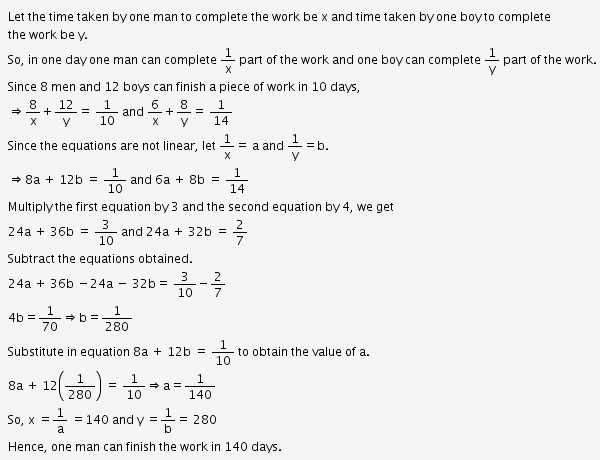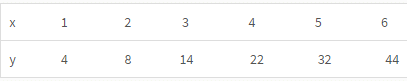# Test: Linear Equations- 2

## 10 Questions MCQ Test IBPS PO Prelims- Study Material, Online Tests, Previous Year | Test: Linear Equations- 2

Description
Attempt Test: Linear Equations- 2 | 10 questions in 10 minutes | Mock test for Banking Exams preparation | Free important questions MCQ to study IBPS PO Prelims- Study Material, Online Tests, Previous Year for Banking Exams Exam | Download free PDF with solutions
QUESTION: 1

### There are 11 more animals than birds in a pet shop. If there are as many birds as animals and if there are as many animals as birds the no. of legs is 4/5 of the original. How many birds and animals are there?

Solution:

Let number of animals be A

Let number of  birds be B.

So first condition we get A=B+11

=>   (11+B)4+2B=X

=>    4B+2(B+11)=4X/5

on solving we get B=11 A=22

Hence (C) is the correct answer.

QUESTION: 2

### 8 girls and 12 boys can finish work in 10 days while 6 girls and 8 boys can finish it in 14 days. Find the time taken by the one girl alone that by one boy alone to finish the work.

Solution:QUESTION: 3

### Mr.Jones gave 40% of the money he had to his wife. He also gave 20% of the remaining amount to his 3 sons.  and half of the amount now left was spent on miscellaneous items and the remaining amount of Rs.12000 was deposited in the bank. How much money did Mr.jones have initially?

Solution:

Let the initial amount be x,

Amount given to his wife =(40/100)x=2x/5

Balance = (x-(2x/5)) = 3x/5

Amount given to his wife = (20/100)*(3x/5) = 3x/25

Balance = 3x/5-3x/25 = 12x/25

Amountt spent on miscellaneous items = (1/2)*(12x/25) = 6x/25 which is equal to 12000

Hence,

=>      6x/25 = 12000

=>      x = 50000

Hence (C) is the correcr answer.

QUESTION: 4

The cost of one pencil, two pens and four erasers is Rs.22 while the cost of five pencils, four pens and two erasers is Rs.32.How much will three pencils, three pens and three erasers cost?

Solution:

Let the cost of 1 pencil, 1 pen and 1 eraser are respectively x, y, z. Then according to the given condition

=>  1x + 2y + 4z = 22

=>  5x + 4y + 2z = 32

On adding these two equations we get

=>   6x + 6y + 6z = 54

=>  3x + 3y + 3z = 27

Hence (C) is the correct answer.

QUESTION: 5

Arun, Akash, Amir and Aswanth go for a picnic. When Arun stands on a weighing machine, Akash also climbs on, and the weight shown was 132 kg. When Akash stands, Amir also climbs on, and the machine shows 130 kg. Similarly the weight of Amir and Aswanth is found as 102 kg and that of Akash and Aswanth is 116 kg. What is Aswanth’s weight?

Solution:

Let weight of arun,akash,amir and aswanth be a,b,c and d respectively.

a+b=132.......(1)

b+c=130.......(2)

c+d=102.......(3)

b+d=116.......(4)

Evaluating 3 and 4 we get

2b=144

b=72

From (4) we have d=116-72=44.

Therefore weight of aswanth is 44 kg.

QUESTION: 6

If a lemon and apple together costs Rs. 12, a tomato and lemon cost Rs.4 and an apple cost of Rs.8 more than a tomato or a lemon, then which of the following can be the price of lemon?

Solution:

Let price of Lemon and Apple is L and A respectively

As given,

=>     L+A=12 .....(1)

=>     L+T=4 .......(2)

=>      A=8+L

=>      or L-A=-8 ......(3)

=>      A=8+T .......(4)
=>      Simply adding eq. 1 and 3

We get..
=>       2L=4

=>       So, L=2

QUESTION: 7

A test has 50 questions. A student scores 1 mark for a correct answer, –1/3 for a wrong answer, and –1/6 for not attempting a question. If the net score of a student is 32, the number of questions answered wrongly by that student cannot be less than

Solution:

Let the no. of questions correctly be x &  no. of questions correctly be y
So, no. of questions left unattempted be (50-x-y)
x - y/3 - (50 - x - y) / 6 = 32
6x - 2y - 50 + x + y = 192
7x - y = 242
y = 7x - 242
If ( x =35 ) y=3
If ( x = 36 ) y=10
So, min. Value of y is 3
The no. of wrongly answered questions cannot be less than 3.

QUESTION: 8

A telecom service provider engages male and female operators for answering 1000 calls per day. A male operator can handle 40 calls per day whereas a female operator can handle 50 calls per day. The male and the female operators get a fixed wage of Rs. 250 and Rs. 300 per day respectively. In addition, a male operator gets Rs. 15 per call he answers and female operator gets Rs. 10 per call she answers. To minimize the total cost, how many male operators should the service provider employ assuming he has to employ more than 7 of the 12 female operators available for the job?

Solution:

There are two equations to be formed 40m + 50f = 1000.

250m + 300f + 40 x 15m + 50 x 10f = A.

850m + 8000f = A.

m and f are the number of males and females. A is the amount paid by the employer.

Then the possible value of f = 8,9,10,11,12.

Iif f= 8,  M =15.

If f = 9,10,11 then m will not be an integer while f =12 then m will be 10.

By putting f = 8 and m=15 , A = 18800.

when f =12 and m= 10 then A = 18100.

Hence, the number of males is 10.

QUESTION: 9In the above table, for suitably chosen constants a, b and c, which one of the following best describes relation between y and x?

Solution:

The data is not linear. So check (2).
Let the equation be y = a + bx + cx.
Putting the values of x and y, we get the following result.
=> 4 = a + b + c,  ....(i)

=> 8 = a + 2b + 4c, and  ...(ii)

=> 14 = a + 3b + 9c. ....(iii)
Solving these, we get a = 2, b = 1 and c = 1.
So the equation is y = 2 + x + x.

QUESTION: 10

If x > 2 and y > -1, then which of the following statements is necessarily true?

Solution:

Use choices. The answer is (2), because –x < –2 and –2 < 2y ⇒ –x < 2y .Use Code STAYHOME200 and get INR 200 additional OFF Use Coupon Code